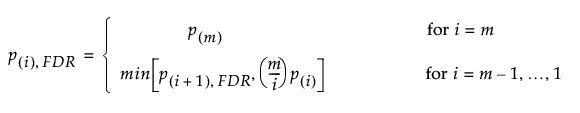Predictive and Specialized Modeling > Response Screening > Statistical Details for the Response Screening Platform
Publication date: 05/24/2021

# Statistical Details for the Response Screening Platform

## The False Discovery Rate

All of the Response Screening plots involve p-values for tests conducted using the FDR technique described in Benjamini and Hochberg (1995). See also Westfall et al. (2011). This method assumes that the p-values are independent and uniformly distributed.

JMP uses the following procedure to control the false discovery rate at level α:

1. Conduct the m hypothesis tests of interest to obtain p-values p1, p2,..., pm.

2. Rank the p-values from smallest to largest. Denote these by p(1)p(2) ≤ ... ≤ p(m).

3. Find the largest p-value for which p(i) ≤ (i/m)α. Suppose this first p-value is the kth largest, p(k).

4. Reject the k hypotheses associated with p-values less than or equal to p(k).

This procedure ensures that the false discovery rate does not exceed α.

The p-values adjusted for the false discovery rate, denoted p(i), FDR, are computed as follows:If a hypothesis has an FDR-adjusted p-value that falls below α, then it is rejected by the procedure.